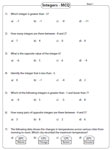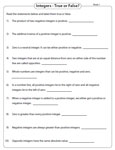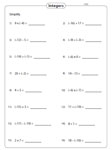# Integer Worksheets

Integer worksheets contain a huge collection of practice pages based on the concepts of addition, subtraction, multiplication, and division. Exclusive pages to compare and order integers and representing integers on a number line are given here with a variety of activities and exercises. These worksheets provide abundant practice for children.

Add or subtract the integers. Simplify the integer equations using addition and subtraction operations. Activity based exercises and word problems are included.

Multiplying and Dividing Integers Worksheets

In these worksheets, multiply and divide to solve the problems. Colorful charts are presented. Interesting exercises and word problems are included.

Comparing and Ordering Integers Worksheets

Learn more about integers by comparing and ordering them. Absolute value and opposite value concepts and MCQs based on it are given for practice in these worksheets.

Integers on a Number Line Worksheets

In these worksheets, represent the integers on a number line, add and subtract integers using number line, writing equations and more.

Integer Worksheets - Mixed Review

Integer worksheets have printable practice sheets to represent them, find the opposite value and absolute values, perform mixed operations, and to identify statements as true or false. Multiple choice questions and simplification of integer expressions are also included here.

Representation of integers

These integer worksheets are based on simple real-life scenarios. Read each statement to represent the integers. Each worksheet has ten statements.Opposite value of integers

Write the opposite value of integers and also mark them on a number line in these worksheets. Three different sections are here for practice.Opposite vs Absolute value

In the first two sections write the opposite value and absolute value of integers. Compare the opposite and absolute value of integers in the third section.Mixed review: Multiple choice questions

Reinforce students' knowledge on integers by practicing these multiple-choice questions. Each worksheet contains seven MCQs.Mixed review: True or false

Ten statements on integer facts are given in these mixed review worksheets. Write true or false against each statement.Mixed operations

Apply all the arithmetic operations to simplify the integer equations. Practice these two levels to get ample understanding of integers.Level 1:

Level 2:

Evaluating integers

In these integer worksheets, substitute the values of the unknown variables in the given expressions and evaluate them.